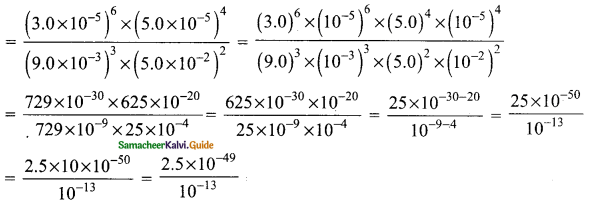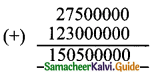Students can download Maths Chapter 2 Real Numbers Ex 2.8 Questions and Answers, Notes, Samacheer Kalvi 9th Maths Guide Pdf helps you to revise the complete Tamilnadu State Board New Syllabus, helps students complete homework assignments and to score high marks in board exams.

## Tamilnadu Samacheer Kalvi 9th Maths Solutions Chapter 2 Real Numbers Ex 2.8

Question 1.
Represent the following numbers in the scientific notation:
(i) 569430000000
(ii) 2000.57
(iii) 0.0000006000
(iv) 0.0009000002
Solution:
(i) 569430000000 = 5.6943 × 1011
(ii) 2000.57 = 2.00057 × 103
(iii) 0.0000006000 = 6.0 × 10-7
(iv) 0.0009000002 = 9.000002 × 10-4Question 2.
Write the following numbers in decimal form:
(i) 3.459 × 106
(ii) 5.678 × 104
(iii) 1.00005 × 10-5
(iv) 2.530009 × 10-7
Solution:
(i) 3.459 × 106
= 3459000
(ii) 5.678 × 104
= 56780
(iii) 1.00005 × 10-5
= 0.0000100005
(iv) 2.530009 × 10-7
= 0.0000002530009Question 3.
Represent the following numbers in scientific notation:
(i) (300000)2 × (20000)4
(ii) (0.000001)11 ÷ (0.005)3
(iii) {(0.00003)6 × (0.00005)4} ÷ {(0.009)3 × (0.05)2}
Solution:
(i) (300000)2 × (20000)4 = (3 × 105)2 × (2 × 104)4
= 32 × (105)2 × 24 × (104)4
= 9 × 1010 × 16 × 1016
= 9 × 16 × 1010-16
= 144 × 1026
= 1.44 × 1028

(ii) (0.000001)11 ÷ (0.005)30.008 × 10-66+9
= 8.0 × 10-3 × 10-57
= 8.0 × 10-3-57
= 8.0 × 10-60

(iii) {(0.00003)6 × (0.00005)4} ÷ {(0.009)3 × (0.05)2}= 2.5 × 10-49+13
= 2.5 × 10-36Question 4.
Represent the following information in scientific notation:
(i) The world population is nearly 7000,000,000.
(ii) One light year means the distance 9460528400000000 km.
(iii) Mass of an electron is 0.000 000 000 000 000 000 000 000 000 00091093822 kg.
Solution:
(i) World population = 7.0 × 109
(ii) Distance = 9.4605 × 1015 km.
(iii) Mass of an electron = 9.1093822 × 10-31 kgQuestion 5.
Simplify:
(2.75 × 107) + (1.23 × 108)
(ii) (1.598 × 1017) – (4.58 × 1015)
(iii) (1.02 × 1010) × (1.20 × 10-3)
(iv) (8.41 × 104) ÷ (4.3 × 105)
Solution:
(i) (2.75 × 107) + (1.23 × 108) = 27500000 + 123000000= 150500000
= 1.505 × 108

(ii) (1.598 × 1017) – (4.58 × 1015) = 1552,20000000000000= 1.5522 × 1017(iii) (1.02 × 1010) × (1.20 × 10-3) = 1.02 × 1.20 × 1010 × 10-3
=1.224 × 107

(iv) (8.41 × 104) ÷ (4.3 × 105) = $$\frac{8.41×10^{4}}{4.3×10^{5}}$$
= $$\frac{8.41}{4.3}$$ × 104-5
= $$\frac{8.41}{4.3}$$ × 10-1
= 1.9558139 × 10-1